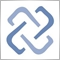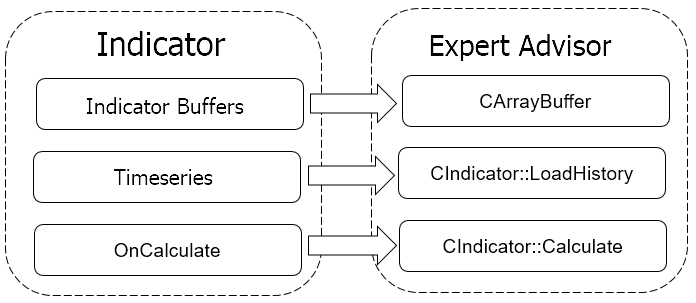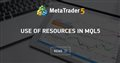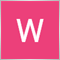# Discussion of article "Implementing indicator calculations into an Expert Advisor code"Moderator
238086

New article Implementing indicator calculations into an Expert Advisor code has been published:

The reasons for moving an indicator code to an Expert Advisor may vary. How to assess the pros and cons of this approach? The article describes implementing an indicator code into an EA. Several experiments are conducted to assess the speed of the EA's operation.

Below is what we are going to do to relocate the indicator calculations to an EA.

1. Arrange the operation of indicator buffers. To do this, create the CArrayBuffer class with methods for data storage and convenient access. Later, create the array of such classes by the number of buffers in the indicator.
2. Relocate the indicator calculation part from the OnCalculate function to the Calculate function of our class.
4. To unify access to the recalculated indicator data, let's create the CopyBuffer function with all necessary parameters in the CIndicator class.

All the work can be visually summarized as follows.Author: Dmitriy Gizlyk86

MetaQuotes:

New article Implementing indicator calculations into an Expert Advisor code has been published:

Author: Dmitriy Gizlyk

Blast from the past here.

Very interesting article indeed! Sound and nice concept... but I fear there is a mistake in the distributed software, and it is not good to leave errors around.

Method GetData. Distributed code is:

```double CIndicator::GetData(const uint buffer_num,const uint shift)
{
if(!Calculate())
return EMPTY_VALUE;
//---
if((int)buffer_num>=m_buffers)
return EMPTY_VALUE;
//---
return ar_IndBuffers[buffer_num].At(m_data_len-shift);
}```

Corrected code should be:

```double CIndicator::GetData(const uint buffer_num,const uint shift)
{
if(!Calculate())
return EMPTY_VALUE;
//---
if((int)buffer_num>=m_buffers)
return EMPTY_VALUE;
//---
return ar_IndBuffers[buffer_num].At(m_data_len-shift - 1);
}```

Array index starts from 0 and last element has index (m_data_len - 1) instead of m_data_len, isn't it?310

rf, section Working with custom indicators of https://www.mql5.com/en/articles/261Use of Resources in MQL5
• www.mql5.com
MQL5 programs not only automate routine calculations, but also can create a full-featured graphical environment. The functions for creating truly interactive controls are now virtually the same rich, as those in classical programming languages. If you want to write a full-fledged stand-alone program in MQL5, use resources in them. Programs with resources are easier to maintain and distribute.9

Hi thanks u somuch